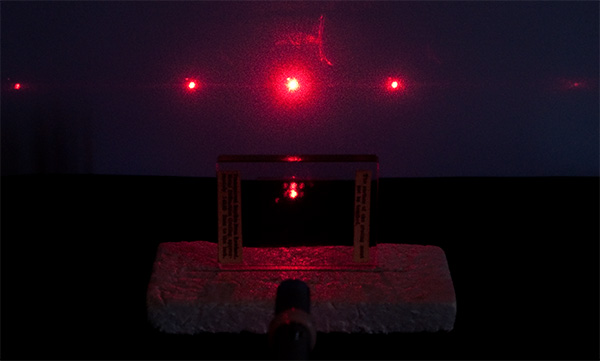# diffraction###### red laser beam passing through a diffraction grating. image: en.academic.ru

Diffraction is a test for wave behaviour.  When a ray of light passes through a diffraction grating, the energy of the incident beam is split into a series of interference fringes.  Constructive interference is occurring at each location where a fringe (or spot) is observed because the rays are in phase when they arrive at these points.###### image: microscopy uk

Find out about diffraction gratings here and here.###### image: laserpointerforums.com

We can measure the relative positions of the fringes in a diffraction pattern to determine the wavelength of the light used.  The diffraction grating equation is$m \lambda = d \sin \theta$

where

• m is the diffracted order  –  some resources may use n instead of m
• λ is the wavelength
• d is the line spacing.

Here is an infrared diffraction experiment you can try at home to calculate the wavelength of the infrared LED in a remote control.

I’ve attached a set of pdf notes and questions on diffraction.  These notes use n rather than m for the diffracted order.

This site uses Akismet to reduce spam. Learn how your comment data is processed.